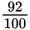# SAT Math Multiple Choice Question 578: Answer and Explanation

### Test Information

Question: 578

8. One function of the Environmental Protection Agency (EPA) is to reduce air pollution. After implementing several pollution reduction programs in a certain city, EPA calculated that the air pollution should decrease by approximately 8% each year. What kind of function could be used to model the amount of air pollution in this city over the next several years, assuming no other significant changes?

• A. A linear function
• C. A polynomial function
• D. An exponential function

Getting to the Answer: Each year, the amount of pollution should be 100 – 8 = 92% of the year before. You can write 92% as, which represents a common ratio from one year to the next. This means that the best model is an exponential function of the form y = a ? (0.92)x.